Courses
Courses for Kids
Free study material
Free LIVE classes
More

# Spring Block Oscillations - JEE Important Topic## What is a Spring-Block Oscillator?

A spring-block oscillator is created by stretching a block of mass m while it is suspended on a vertical spring and allowing it to bounce back and forth. An illustration of the simple harmonic motion is this bouncing. Three different types of energy interact in a spring-block oscillator: kinetic energy in the middle, elastic potential energy at the bottom, and gravitational potential energy at the top. This makes it a little bit more complex than a pendulum or a horizontal spring in terms of basic harmonic motion.

## Simple Harmonic Motion (SHM)

Simple harmonic motion is any motion in which the displacement is reversed by an opposing restoring force that is proportional to the displacement. Or, to put it another way, the more you pull it, the more it wants to turn around and head back in the direction of the centre. An illustration of this is the spring-block oscillator, which exerts greater force back toward the equilibrium position the more you stretch it.

## Motion of Spring

The oscillation of a mass on a spring when it is subject to the linear elastic restoring force specified by Hooke's law is a good example of simple harmonic motion, which may be used as a mathematical model for many different motions. The motion has a single resonant frequency and is sinusoidal in time.

## What is the Spring Block System?

Simply put, a spring system with a block hung or fastened to the free end is known as a spring-block or spring-mass system. The period of any item moving in a simple harmonic motion may typically be determined using the spring-mass system. There are numerous other applications for the spring-mass system. For instance, the spring-mass system can be used to replicate foot skin deformation and the action of human tendons using computer images.

Creating an equation between force and displacement or between acceleration and displacement is the first stage. Then, we use a common expression to calculate the frequency and time period. In general, we'll be analysing the following versions of a block and spring(s):

• Horizontal Block – Spring System

• Vertical Block – Spring System

• Block connected to springs in series

• Block in between two springs

• Block connected to springs in parallel

## Frequency of Spring-Block System

The time it takes an object to complete one oscillation and return to its initial position is measured in terms of a period, or T. The angular frequency ω is given by $\omega =2\pi /T$ .

## Time Period of a Spring-Block System

In a closed environment, a spring with mass m and spring constant k exhibits a straightforward harmonic motion. The Spring force is given by

F = -kx

And

$a=-{{\omega }^{2}}x$

where k = spring’s constant, x = displacement, a = acceleration, and $w=$angular velocity  in SHM.

Now, from Newton’s law of motion, the force is given by

F = ma

So, equating them,

$-m{{\omega }^{2}}x=-kx$

$\Rightarrow k=m{{\omega }^{2}}$

$\omega =\sqrt{\frac{k}{m}}$

Now, the time period is given by

$T=\frac{2\pi }{\omega }$

$\therefore T=2\pi \sqrt{\frac{m}{k}}$

It is obvious from the above equation that neither gravitational acceleration nor amplitude affect the oscillation's period. Furthermore, the period of oscillation cannot be changed by a constant force. While this is happening, the length of time directly relates to the mass of the body that is attached to the spring. If you attach a hefty object to it, it will oscillate more slowly.

## Horizontal Block-Spring System

The ideal spring is governed by Hooke's law, which relates spring force to displacement as follows:

$F=-kx$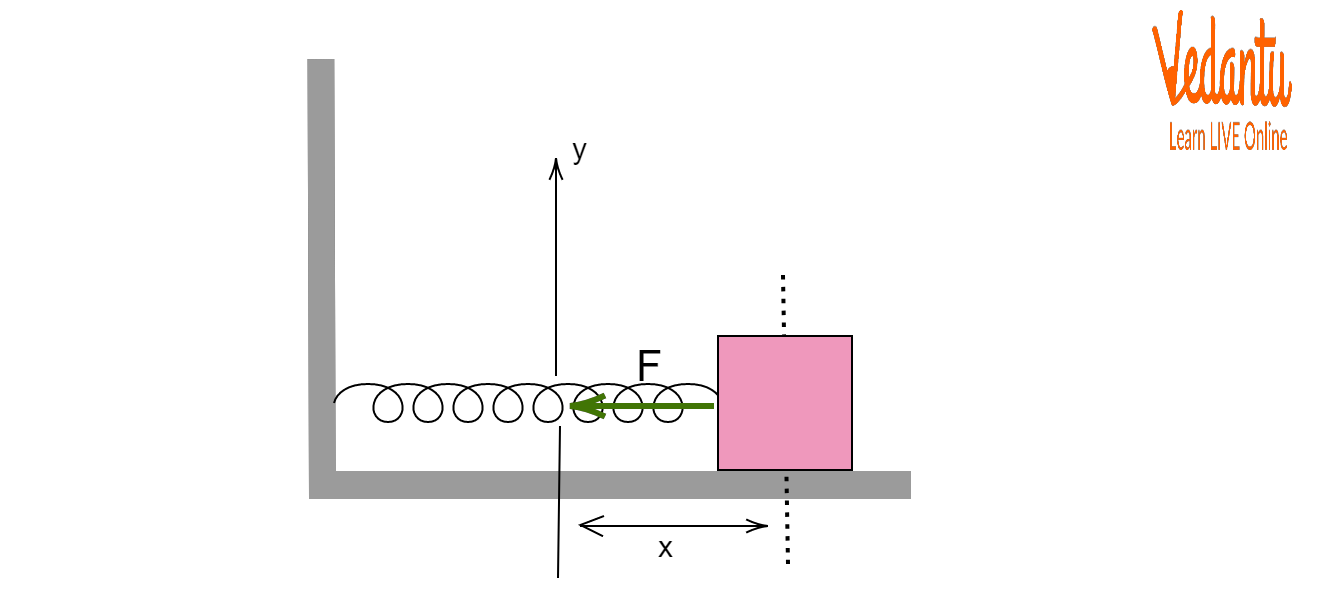Horizontal Block-Spring System

## Vertical Block-Spring System

Gravitational force is applied differently in a vertical block-spring system than it is in a horizontal layout. Gravitational force is not taken into account for the study because it is perpendicular to motion in a horizontal orientation. However, in the vertical orientation, the spring is expanded because of the block's weight before the block is positioned in SHM. In the expanded position, it is balanced between the forces of gravity and the spring.

It is obvious that the equilibrium point is the oscillator's centre. Instead of oscillating around the position of neutral spring length as in the case of a horizontal arrangement, the block oscillates about the expanded position. Let us consider that the spring is extended by a vertical length ${{y}_{0}}$ from a neutral position when it is in the equilibrium position. The spring force acting on the block for an additional extension "y" of the spring is equal to the product of the total displacement from the neutral position and the spring constant.

$F=-k({{y}_{0}}+y)$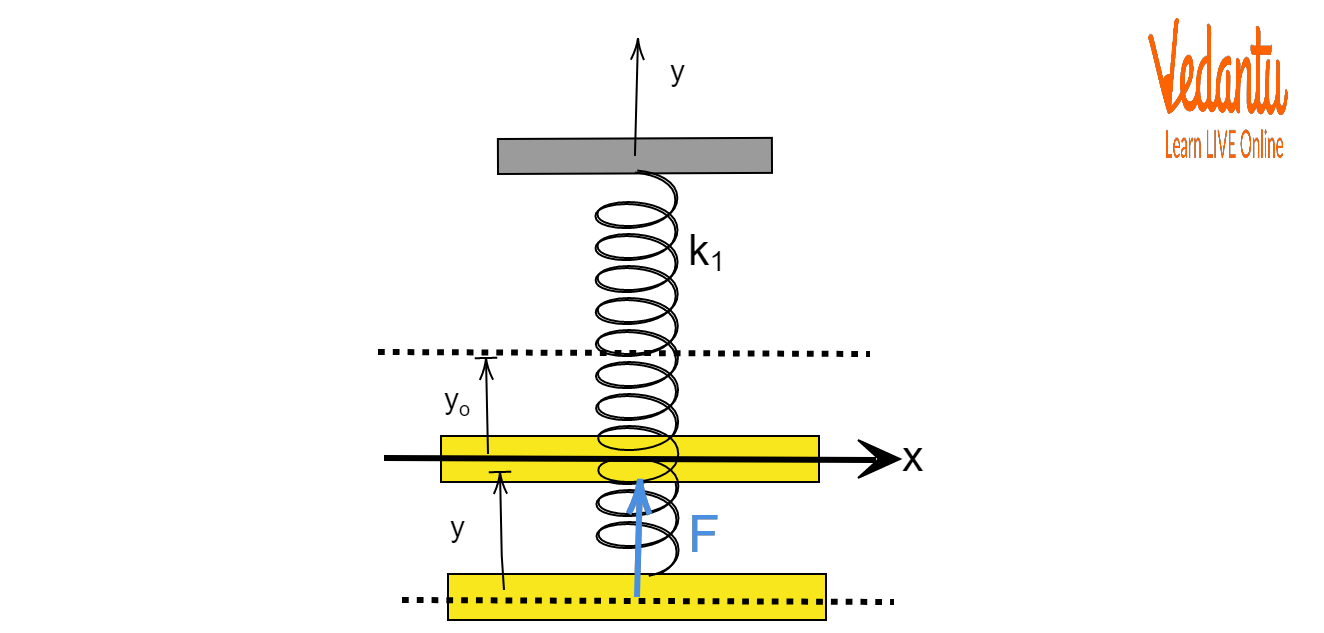Vertical Block-Spring System

## Block Connected to Springs in Series

We take into account two springs with various spring constants. When an outside force, such as gravity, acts, both springs elongate at once. Since springs have no mass, their forces are the same throughout two springs. Due to the varied spring stiffness, this force causes two springs to elongate in distinct ways. Let ${{y}_{1}}$ and ${{y}_{2}}$ be the elongations in two springs. The net restoring force for each of the springs is stated as follows, just as it was for the single spring:

$y={y_1}+ {y_2}$

Since $F=-ky$, so we can write

$\Rightarrow -\dfrac{F}{k_{net}}=-\dfrac{F}{k_{1}}-\dfrac{F}{k_{1}}$

$\Rightarrow \dfrac{1}{k_{net}}=\dfrac{1}{k_1}+\dfrac{1}{k_1}$

$\Rightarrow k_{net}=\dfrac{k_1 k_2}{k_1 + k_2}$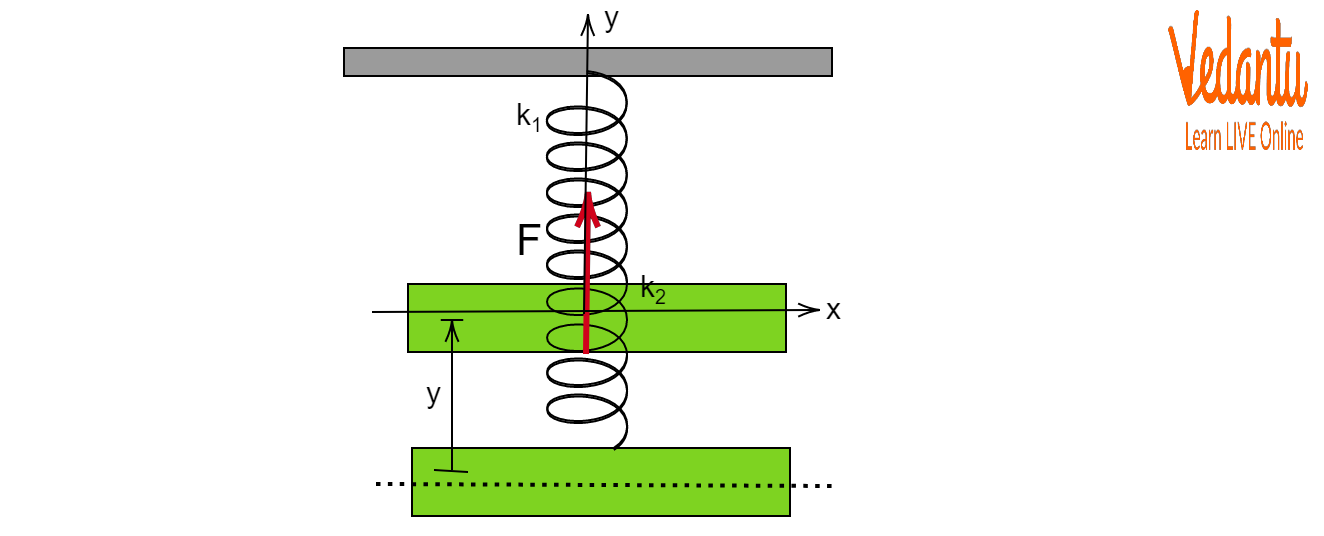Block Connected to Springs in Series

## Block in between Two Springs

According to the figure, this setup involves tying a block between two springs. We study oscillation from the reference position of equilibrium in order to analyse oscillation. Allow a tiny displacement of the block in a downward direction (reasoning is similar if the block is displaced upward). While the lower spring is compressed, the top spring is stretched. The upward motion is the direction of the spring forces caused by any of the springs. The net downward displacement and the net restoring force are connected as follows:

${{F}_{net}}=-{{k}_{1}}y-{{k}_{2}}y=-({{k}_{1}}+{{k}_{2}})y$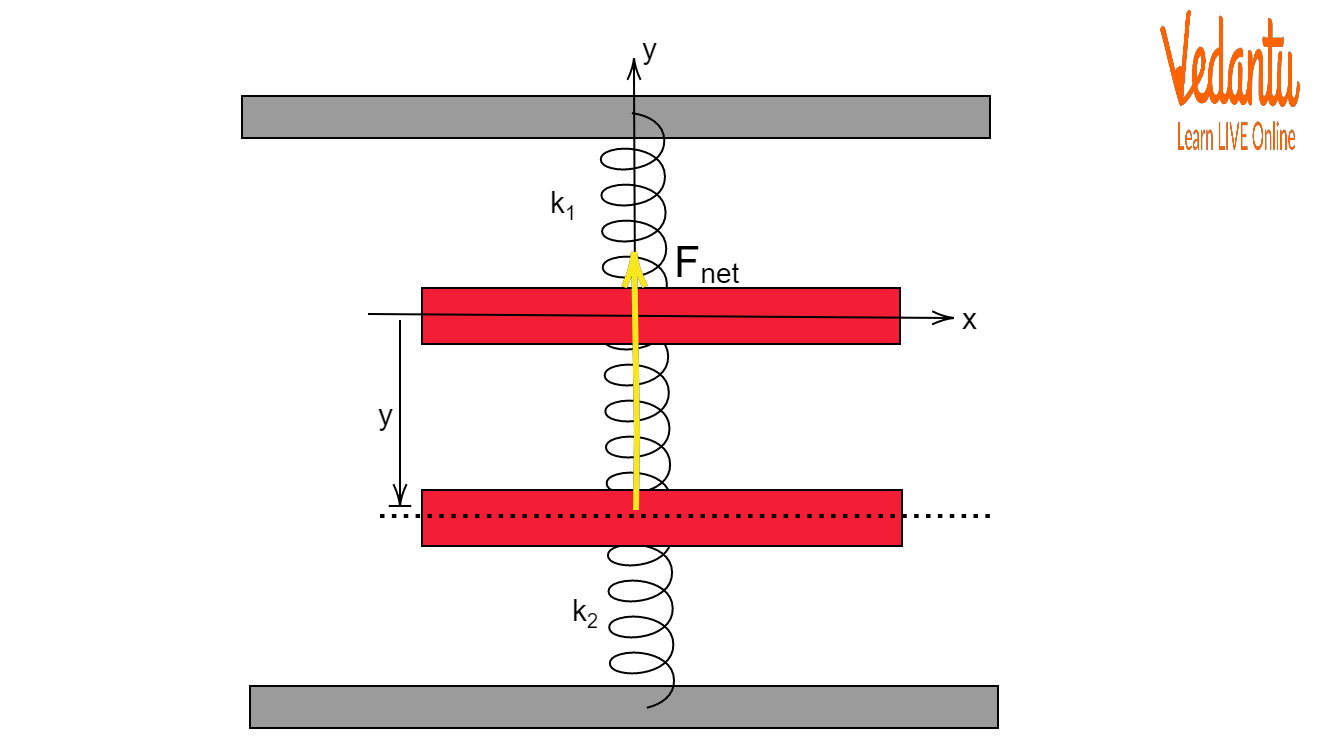Block in between Two Springs

## Block Connected to Springs in Parallel

Here, we take into account a block that is supported horizontally by two parallel springs with various spring constants, as seen in the image. The block oscillates around the equilibrium position when it is slightly pulled. The following is the block's net restoring force:

${{F}_{net}}=-{{k}_{1}}y-{{k}_{2}}y=-({{k}_{1}}+{{k}_{2}})y$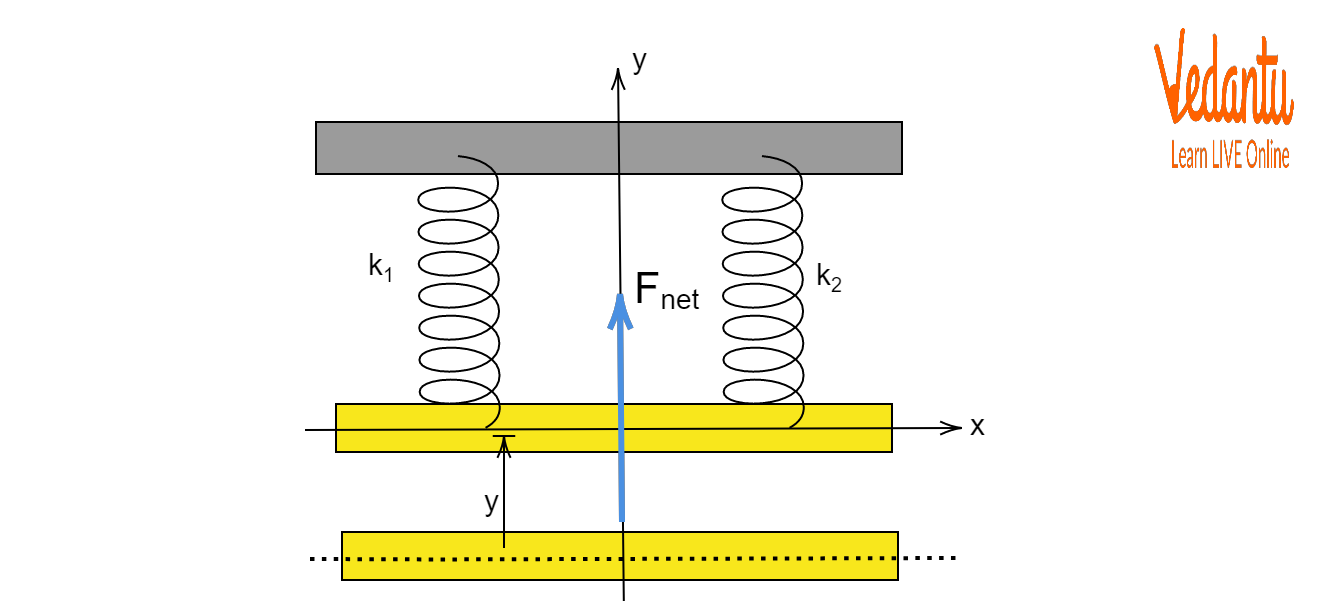Block Connected to Springs in Parallel

## Conclusion

In a simple harmonic motion, the system's acceleration and, consequently, the net force act in the opposite directions of the displacement and are proportional to the displacement. Simply said, a spring-mass system is a spring system with a block hung or fastened to the free end of the spring. The period of any item moving in a simple harmonic motion may typically be determined using the spring-mass system.

Last updated date: 30th May 2023
Total views: 88.8k
Views today: 1.47k

## FAQs on Spring Block Oscillations - JEE Important Topic

1. Why does increasing the amplitude have no net effect on the period of the oscillation?

In an oscillating system, the amplitude is the amount by which the oscillating variable changes with each oscillation. It is the greatest deviation an object makes from its equilibrium position when it is oscillating around it. As the amplitude rises, the mass moves farther with each cycle. The restoring force, however, likewise rises as the amplitude does. The acceleration of the mass increases proportionally with the increase in force, causing the mass to travel farther in the same amount of time. Therefore, increasing the amplitude has no overall impact on the oscillation's period.

2. Explain briefly Simple Harmonic Motion (SHM).

Simple harmonic motion is any motion in which the displacement is reversed by an opposing restoring force that is proportional to the displacement. Or, to put it another way, the more you pull it, the more it wants to turn around and head back in the direction of the centre. An illustration of this is the spring-block oscillator, which exerts the greater force back toward the equilibrium position the more you stretch it. The acceleration of the particle at any position is directly proportional to the displacement from the mean position, and it can be thought of as an oscillatory motion. This particular oscillatory motion is a special case.